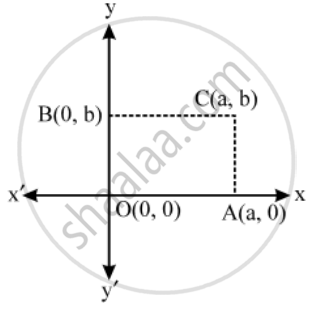# Prove that the diagonals of a rectangle bisect each other and are equal. - Mathematics

Sum

Prove that the diagonals of a rectangle bisect each other and are equal.

#### Solution

Let OACB be a rectangle such that OA is along x-axis and OB is along y-axis. Let OA = a and OB = b.Then, the coordinates of A and B are (a, 0) and (0, b) respectively. Since, OACB is a rectangle. Therefore,

AC = Ob ⇒ AC = b

Thus, we have

OA = a and AC = b

So, the coordiantes of C are (a, b).

The coordinates of the mid-point of OC are

( \frac{a+0}{2},\ \frac{b+0}{2})=( \frac{a}{2},\frac{b}{2})

Also, the coordinates of the mid-points of AB are

( \frac{a+0}{2},\ \frac{0+b}{2})=( \frac{a}{2},\frac{b}{2})

Clearly, coordinates of the mid-point of OC and AB are same.

Hence, OC and AB bisect each other.

OC\text{ }=sqrt((a-0)^{2}+(0-b)^{2})=\sqrt(a^{2}+b^{2})

AB\text{ }=sqrt((a-0)^{2}+(0-b)^{2})=\sqrt(a^{2}+b^{2})

∴ OC = AB

Concept: Section Formula
Is there an error in this question or solution?

Share